3.MD.7.C.-Test1B Show the area of a rectangle with whole-number side lengths
 Name:    3.MD.7.C.-Test1B Show the area of a rectangle with whole-number side lengths

Multiple Choice
Identify the choice that best completes the statement or answers the question.

1.

Which number sentence would solve the area for this rectangle?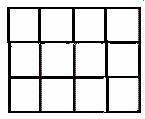a. none of these c. 12 = 4 x 3 b. 4 = 12 x 3 d. 3 = 12 x 4

2.

Which number sentence would show how to solve for the area of this rectangle?a. none of these c. 6 x 6 = 36 b. 6 x 5 = 30 d. 6 x 4 = 24

3.

Which number sentence would show how to solve for the area of this rectangle?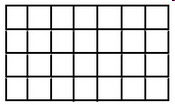a. none of these c. 4 = 28 x 7 b. 7 = 4 x 28 d. 28 = 7 x 4

4.

Which number sentence would show how to solve for the area of this rectangle?a. none of these c. 20 = 4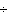5 b. 4 = 20 x 5 d. 5 = 20 x 4

5.

Which number sentence would show how to solve for the area of this rectangle?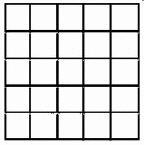a. 5 x 5 = 25 c. none of these b. 25 x 5 = 5 d. 5 x 4 = 25

6.

To find the area of a rectangle you multiply length and __________.
 a. weight c. width b. mass d. height

7.

What number sentence would solve for the area of this rectangle?a. 6 x 3 = 18 c. 6 x 2 = 12 b. none of these d. 2 x 3 = 6

8.

What number sentence would solve for the area of this rectangle?a. 4 = 24 x 6 c. 6 = 24 x 4 b. 24 = 6 x 4 d. none of these

9.

What number sentence would solve for the area of this rectangle?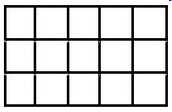a. 15 = 5 x 3 c. 3 = 15 x 5 b. 5 = 15 x 3 d. none of these

10.

To find area you need to multiply width and ______________.
 a. density c. volume b. mass d. length